Working with Matrices
 Matrices are rectangular arrays of elements.  The dimension of a matrix is the number of rows by the number of columns.

Adding Matrices - matrices must be of the same dimension to be added.
Add: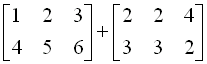First Enter the Matrices (one at a time):

Step 1:  Go to Matrix
(above the  x-1 key)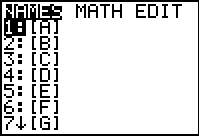If dimensions appear next to the names of the matrices, such as 3x3, a matrix is already stored in the calculator.  You may save it by moving to a new name, or overwrite it.

Step 2:  Arrow to the right to
EDIT to allow for
entering the matrix.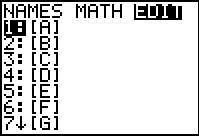Step 3:  Type in the dimensions (size) of your matrix and enter the elements (press ENTER).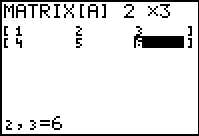Step 4:  Repeat this process for
the second matrix

.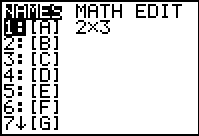Step 5: Arrow to the right to
EDIT and choose a
new name.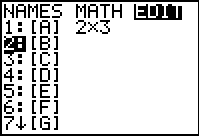Step 6:  Type in the dimensions (size) of your matrix and enter the elements (press ENTER).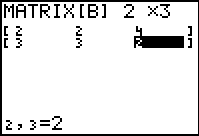Now, add:
Step 7:
Return to the home
screen.  Go to Matrix

to get the names of the
matrices for adding.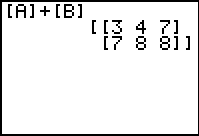The answer to the addition, as seen on the calculator screen,
is =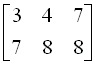Multiplying Matrices- for multiplication to occur, the dimensions of the matrices must be related in the following manner:  m x n  times  n x r  yields  m x r
Multiply: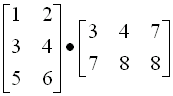First Enter the Matrices (one at a time) as shown above:

Step 1:   Once the matrices are entered, you should see their dimensions in residence when you go to Matrix  (above the  x-1 key)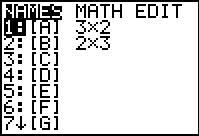Step 2:   Return to the home
screen.  Go to Matrix

to get the names of the
matrices for multiplying.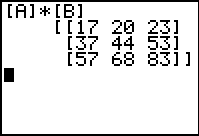The product, as seen on the calculator screen,
is =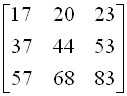Using Matrices to Solve Systems of Equations:

1.  (using the inverse coefficient matrix)
Write this system as a matrix equation and solve:  3x  + 5y = 7 and 6x - y = -8

Step 1:  Line up the x, y and
constant values.

3x  + 5y =  7
6x   -   y = -8

Step 2:  Write as equivalent
matrices.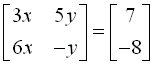Step 3:  Rewrite to separate out
the variables.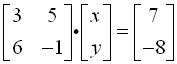Step 4:  Enter the two numerical matrices in the calculator.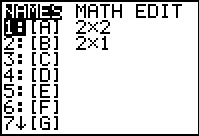Step 5:  The solution is obtained by multiplying both sides of the equation by the inverse of the matrix which is multiplied times
the variables.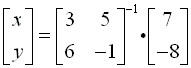Step 6:  Go to the home screen and enter the right side of the previous equation.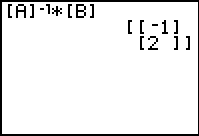The answer to the system, as seen on the calculator screen,
is  x = -1 and y = 2.

Note: If the determinant of a matrix is zero, the matrix does not have an inverse. Thus, a single point, (x,y), solution cannot be found.

2.  (using Gauss-Jordan elimination method with reduced row echelon form )

Solve this system of equations:
 2x - 3y + z = -5 4x -  y - 2z = -7 -x + 2z = -1
 Step 1:  Line up the variables and               constants      2x - 3y  + z = -5      4x -  y  - 2z = -7      -x + 0y + 2z = -1 Step 2:  Write as an augmented               matrix and enter into               calculator.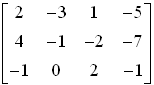Step 3:  From the home screen, choose the rref function.  [Go to Matrix  (above the  x-1key), move right→MATH, choose B: rref]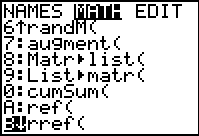Step 4:  Choose name of matrix              and hit ENTER.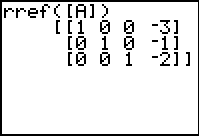Step 5:  The answer to the system, will be the last column on the calculator screen:           x = -3           y = -1           z = -2.Finding Your Way Around TABLE of CONTENTS